Next: Piston-Generated Expansion Wave Up: One-Dimensional Compressible Inviscid Flow Previous: Normal Shocks

# Piston-Generated Shock Wave

Consider the situation illustrated in Figure 14.2 in which, at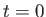, a tight-fitting piston is suddenly pushed into a stationary gas, contained in a uniform tube, at the steady speed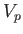, generating a shock front that propagates away from the piston, and into the gas, at the speed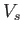. Suppose that the piston is located at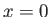at, and moves in the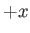-direction. The gas can be divided into two regions. In region, which lies to the right of the shock wave (i.e.,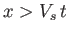), the gas remains undisturbed. Hence, its velocity is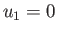. Let its pressure, density, and temperature be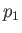,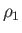, and, respectively. In region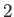, which lies between the piston and the shock wave (i.e.,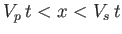), the gas is co-moving with the piston, so its velocity is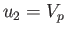. Let its pressure, density, and temperature, be,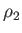, and, respectively. Thus, the system is formally the same as that discussed in the final paragraph of the previous section, provided that we make the identifications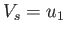and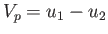.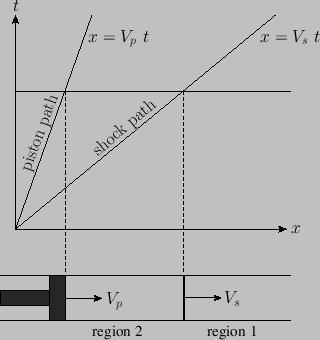Let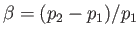be our conventional measure of shock strength. It follows from Equations (14.111) and (14.112) that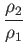(14.127)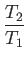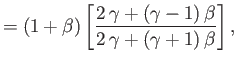(14.128)

where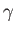is the gas's ratio of specific heats. We can define the Mach number of the shock wave as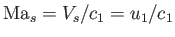, where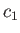is the sound speed in the unperturbed gas. According to Equation (14.110),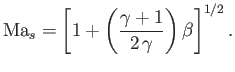(14.129)

Finally, we can define the Mach number of the piston as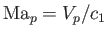. Thus,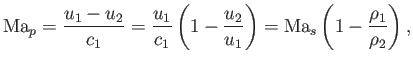(14.130)

where use has been made of Equation (14.111). Equations (14.127) and (14.129) can be combined with the previous equation to give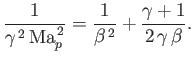(14.131)

Thus, Equations (14.127)-(14.129), and (14.131), fully determine the parameters of the shocked gas, as well as the speed of the shock front, in terms of the parameters of the unperturbed gas and the piston speed. In particular, for a weak shock (i.e.,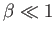),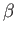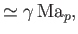(14.132)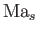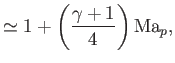(14.133)

whereas for a strong shock (i.e.,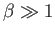),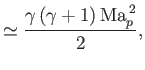(14.134)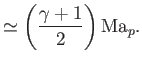(14.135)

Thus, a weak shock is associated with a subsonic piston (i.e.,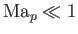), whereas a strong shock is associated with a supersonic piston (i.e.,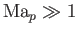).Next: Piston-Generated Expansion Wave Up: One-Dimensional Compressible Inviscid Flow Previous: Normal Shocks
Richard Fitzpatrick 2016-03-31